#### How to Calculate Overtime Hours in Excel

Calculating overtime hours in Excel is indeed a useful skill, especially for HR and payroll professionals. The calculation of work overtime is dependent on knowing when an employee starts and when he or she finishes for the day.

Let’s start by laying out the data. Create a worksheet with columns for the start time, end time, and total hours worked each day. You can also include columns for the normal workweek hours and the overtime hours.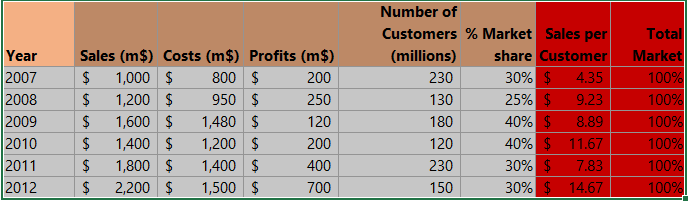## Overtime formula

1. Click under Total (F2) (1), and type in =(E6-B6 + (E6<B6))*24 (2).

You can use conditional formatting to highlight cells containing overtime hours or to apply different formatting rules based on specific conditions. This makes it easier to identify overtime periods.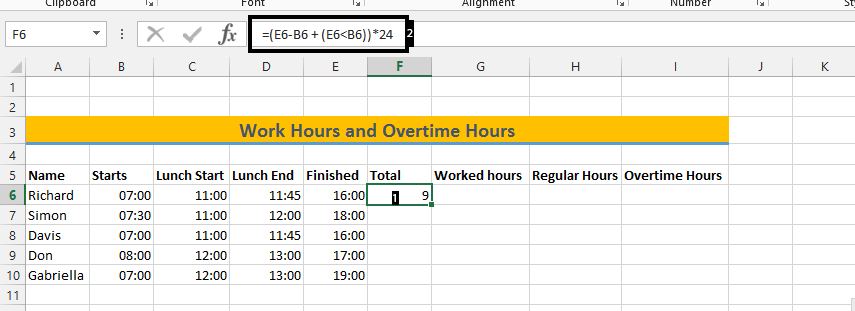2. Click on G6 (under worked hours) (1), and type =((E6-B6+(E6<B6))-(D6-C6+(D6<C6)))*24.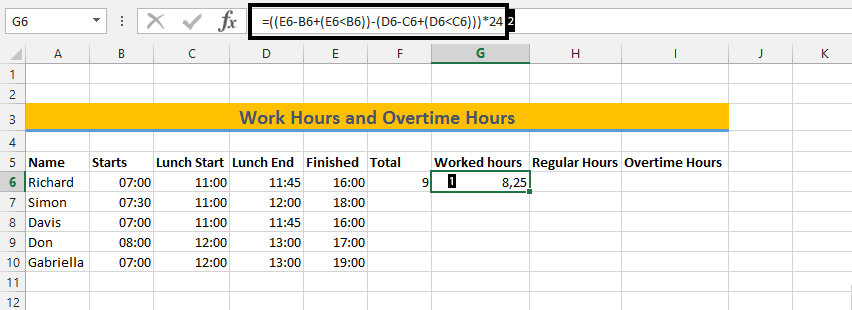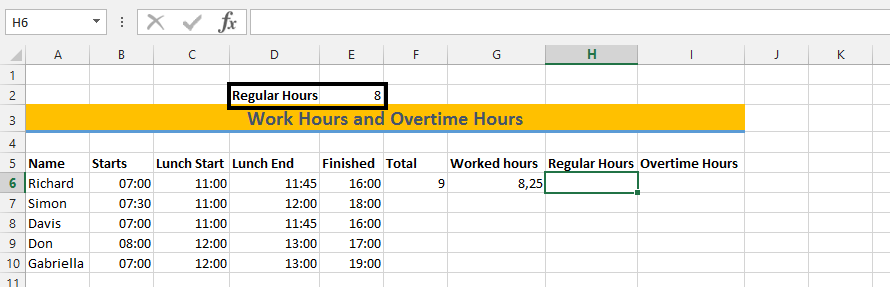4. Click on H6 (under regular hours) (1), and type =Min(E2;G6), then press enter.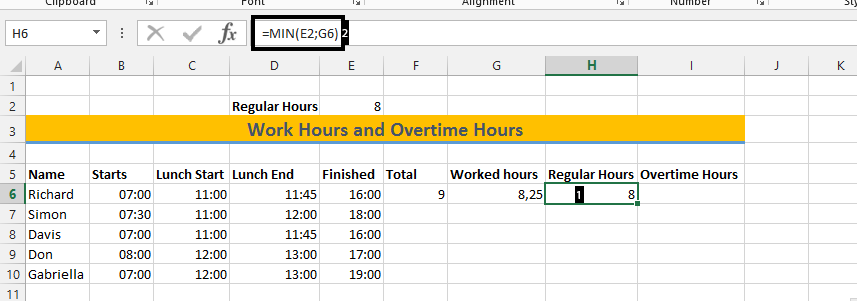## Overtime calculator

In Excel, time is treated as fractions of a day. For example, 1 hour is equivalent to 1/24 of a day. To calculate time differences, it’s essential to grasp this concept.

5. Click on I6 (under Overtime Hours) (1), and type =MAX(0;G6-\$E\$2), then press enter.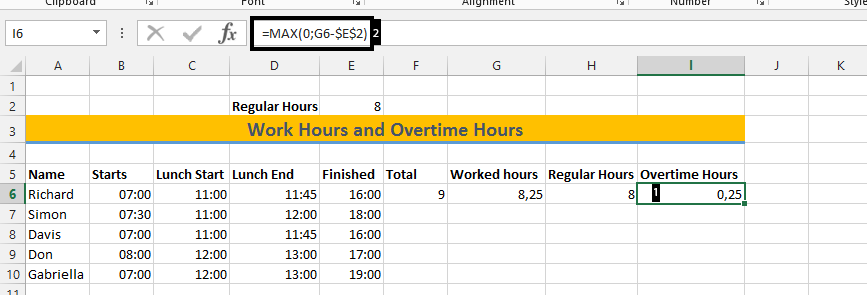6. Mark from F6 to I6 (1), and double click on the small square (2).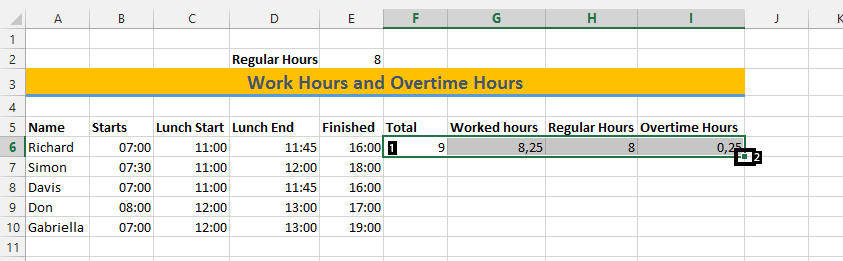If there is anything that does not show correctly, you should right-click on that cell, choose Format Cells, and then click on the number, and finally press Okay.

By using these formulas, you can easily calculate the overtime hours for a set of data in Microsoft Excel. You can also use conditional formatting and other Excel features to further analyze and visualize the data to draw conclusions and make informed decisions.